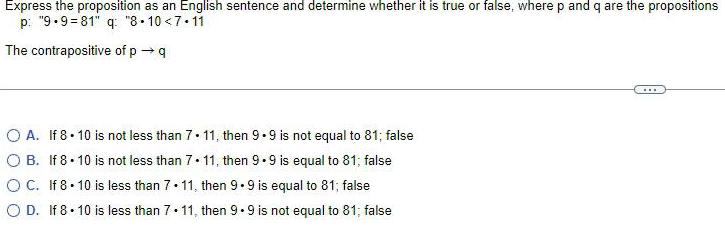Question:

# Express the proposition as an English sentence and determine

Last updated: 7/5/2022Express the proposition as an English sentence and determine whether it is true or false, where p and q are the propositions p: "9.9=81" q: "8.10<7.11 The contrapositive of p →q A. If 8.10 is not less than 7. 11, then 9.9 is not equal to 81; false B. If 8.10 is not less than 7. 11, then 9.9 is equal to 81; false C. If 8.10 is less than 7.11, then 9.9 is equal to 81; false D. If 8.10 is less than 7.11, then 9.9 is not equal to 81; false